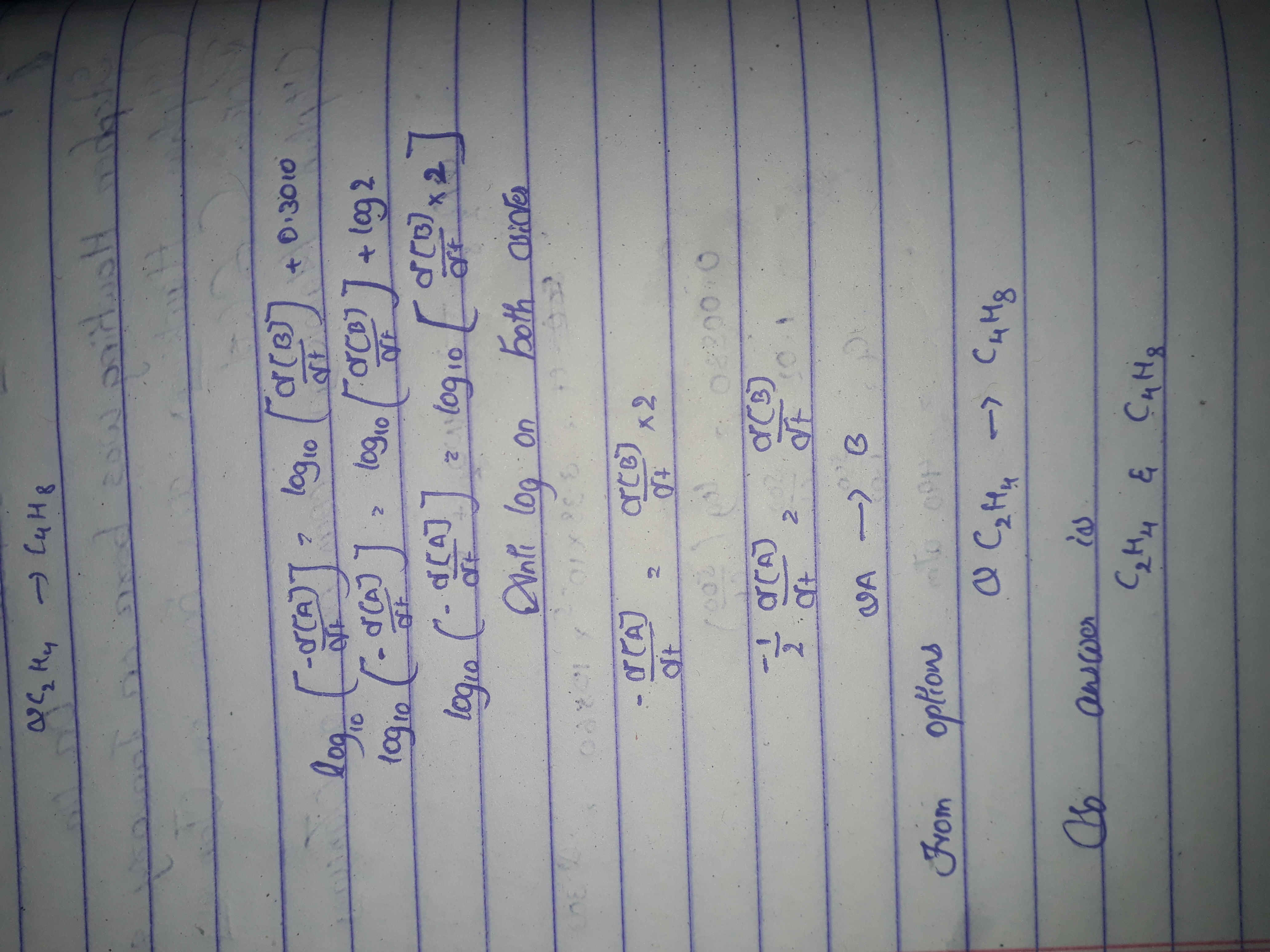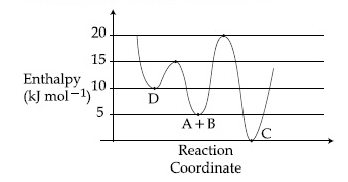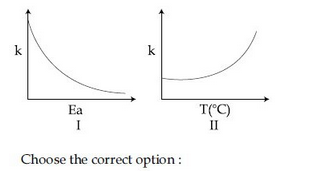## Filters

Sort by :
Clear All
Q

In the following reaction;

'A' and 'B' respectively can be :

• Option 1)

and

• Option 2)

and

• Option 3)

and

• Option 4)

n-Butane and Iso-butaneA bacterial infection in an internal wound grows as ,

where the time t is in hours. A dose of antibiotic, taken orally, needs 1 hour to reach the wound.

Once it reaches there, the bacterial population goes down as .

What will be the plot of  vs. t after 1 hour ?

• Option 1)• Option 2)• Option 3)• Option 4)From o to 1 hour =>  After 1 hour, it is given :   at t = 1 hour ,  Now, This equation is similar to the straight line equation (Y=mx+C) So, the curve will be straight line. option (3) is correct.      Option 1)Option 2)Option 3)Option 4)

For the reaction of  with , the rate constant is  at  and  at  The activation energy for the reaction , in  is :

• Option 1)

• Option 2)

• Option 3)

• Option 4)

Given                                      Option 1)Option 2)Option 3) Option 4)

Consider the given plot of enthapy of the following reaction between A and B .

Identify the incorrect statement .• Option 1)

Activation enthapy to form C is  less than that to form D.

• Option 2)

C is the thermodynamically stable product.

• Option 3)

D is kinetically stable product .

• Option 4)

Formation of A and B from C has highest enthaply of activation .

Activation Energy - The minimum kinetic energy that reactants must have in order to form products. - wherein Description: E.g.: In a gas phase reaction numerous collisions take place but only a tiny proportion which has sufficient energy to cross the energy barrier leads to product formation.           EC (activation energy of C) = (20-5)kJ mol- = 15 kJ mol- ED ( activation energy of D) =...

The given plots represent the variation of the concrntration of a reactant  with time for two different reactions (i) and (ii). The respective orders of the reactions are :• Option 1)

• Option 2)

• Option 3)

• Option 4)

=Concentration of reactant at  time 1st  order :                                                          Zero order                  (negative) So,order are  respectively            Option 1) Option 2) Option 3) Option 4)

For a reaction schemeif the rate of formation of B is set to be zero then the concentration of B is given by:

• Option 1)

• Option 2)

• Option 3)

• Option 4)

(given) [B]=?               net rate of formation of  Option 1)Option 2)Option 3)Option 4)

For the reaction , the values of initial rate at different reactant concentrations are given in the table below. The rate law for the reaction is :

 0.05 0.05 0.045 0.10 0.05 0.090 0.20 0.10 0.72

• Option 1)

• Option 2)

• Option 3)

• Option 4)

Rate law 0.05 0.05 0.045 0.10 0.05 0.090 0.20 0.10 0.72                Option 1) Option 2) Option 3)   Option 4)

required for a reaction is produced by the decomposition of  in  as per the equation, .

The initial concentration of  is  and it is  after 30 minutes. The rate of formation of  is:

• Option 1)

• Option 2)

• Option 3)

• Option 4)

()                   so,  from the reaction    Option 1)       Option 2) Option 3) Option 4)
how to find slowest reaction in chemical kinetic
The reaction mechanism is the step-by-step process by which reactants actually become products. The overall reaction rate depends entirely on the rate of the slowest step. If the first step is the slowest, entire reaction must wait for it, then it is the rate-determining step.
Screenshot_1196.pngE Online Coaching for IIT JEET X E Online Coaching for IIT JEET X E Online Coaching for IIT JEE C a https://mail.google.com/mail/u/O/?tab=rm#inbox?projector= 1 (4) Cant be concluded about shift direction Online Coaching for IIT JEE X M Inbox (176) - nelantisanthos x F 28. 31. 24-02-2019 Sup A conductor which is shaped as shown in the figure, carries a current l. The magnetic field at the point O (2) (3) (4) = am p is (O, b, â€”c) aj, P is (â€”b, O, â€”c) a), P is (b, O, â€”c) 30. If the frequency of light in a photoelectric experiment is doubled, the stopping potential will be (1) (2) (3) More than double (4) Less than double [PART-B : CHEMISTRY] For first order reaction, NO(g) the half life is 12 min. How much time is required to reduce [NO] to 20% of initial concentration? 32. How many grams of Ag are deposited by passing 1.93 A current for 5 minutes through AgN03(aq) solution? (Atomic weight Of Ag = 108) (1) 0.648 g (3) 0.45 g (2) 0.94 g (4) 1.10 g (2) (3) (4) 24 min 28 min 18 min 32 min Page 5 33. (5) 19 Solubility Of BaF2 in water at certain temperature is 0.02 M. The solubility product of BaF2 is (1) 3.2 x 10-5 (3) 8 x 104 (2) 6 x 10-6 Activate Vindows 'gs to activate Windows.
The time required to reduce concentration of NO can be calculated as:

K reprsent the rate of reaction when log K is ploted against 1/T the graph is obtain as

Let us understand this question with the help of a graph plotted between log K and 1/T

The rate of a reaction A doubles on increasing the temperature from 300 to 310 K.  By how much, the temperature

• Option 1)

9.84 K

• Option 2)

4.92 K

• Option 3)

2.45 K

• Option 4)

19.67 K

Option 1) 9.84 K Option 2)  4.92 K Option 3)  2.45 K Option 4) 19.67 K

A reaction having equal energies of activation for forward and reverse reactions has:

• Option 1)H =G =S=0

• Option 2)S = 0

• Option 3)G = 0

• Option 4)H = 0

Option 1) H = G = S=0 Option 2) S = 0 Option 3) G = 0 Option 4) H = 0

If a reaction follows the Arrhenius equation , the plot Ink vs  gives straight line with a gradient  (-y) unit . The energy required to activate the reactant is:

• Option 1)

-y unit

• Option 2)

y/R unit

• Option 3)

y unit

• Option 4)

yR unit

Arrhenius Parameters - The pre-exponential factor/ frequency factor (A) and activation energy () are called Arrhenius parameters. - wherein Formula: A = Pre-exponential factor  = Activation Energy       Arrhenius Equation - Arrhenius gave the quantitative dependence of rate constant on temperature by the Arrhenius equation. - wherein = Rate constant aas we know that Arrhenius equation...

The reaction 2X  B is a zeroth order reaction. If the initial concentration of X is 0.2 M , the half- life is 6 h. When the initial concentration of X is 0.5 M, the time required to reach its final concentration of 0.2 M will be :

• Option 1)

7.2 h

• Option 2)

9.0 h

• Option 3)

18.0 h

• Option 4)

12.0 h

3

For a reaction, consider the plot of In k versus 1/T given in the figure. If the rate constant of this reaction at 400 K is 10-5 s-1 , then the rate constant at 500 K is :• Option 1)

• Option 2)

• Option 3)

• Option 4)

Chemical Kinetics - The branch of chemistry which predicts the rate and mechanism of a process -     Effect of Temperature and Activation Energy on rate constant -                                   decreases with the increase in T                   increases with increase in T  = Activation Energy T = Temperature   = Rate constant - wherein       Rate of reaction - The  rate of the reaction...

For an elementry chemical reation the expression for  is:

• Option 1)

• Option 2)

• Option 3)

• Option 4)

Rates in presence of stoichiometry of reactants/products - When stoichiometry coefficients of reactants/ products are not equal to one, the rate of disappearance of  & the rate of appearance of products is divided by their respective stoichiometric coefficients - wherein    Option 1)Option 2)Option 3)Option 4)

Consider the given plots for a reaction obeying Arrhenius equation ( ) : (k and are rate constant and activation energy , respectively )• Option 1)

I is right but II is wrong .

• Option 2)

Both I and II are correct.

• Option 3)

I is wrong but II is right .

• Option 4)

Both I and II are wrong

Arrhenius Equation - Arrhenius gave the quantitative dependence of rate constant on temperature by the Arrhenius equation. - wherein = Rate constant as we have learned in Arrhenius equation when we increase Ea, K should decrease    Option 1)I is right but II is wrong .Option 2)Both I and II are correct.Option 3)I is wrong but II is right .Option 4)Both I and II are wrong

Decomposition of X exhibits a rate constant of 0.05 . How many years are required for the decomposition of 5  of X into 2.5  ?

• Option 1)

25

• Option 2)

40

• Option 3)

20

• Option 4)

50

Rate Determining Step - The overall rate of reaction is controlled by the slowest step in a reaction called the rate determining step. - wherein e.g. As wehave learned in zero order reaction unit of rate constant it is zero order reaction then half life of the reaction will be  Option 1)25Option 2)40Option 3)20Option 4)50

For the reaction ,  products , when the concentrations of A and B both were doubled , the rate of the reaction increased from  . When the concentration of A alone is doubled , the rate increased from .

Which one of the following statement is correct ?

• Option 1)

Order of the reaction with respect to B is 1

• Option 2)

Order of reaction with respect to A is 2

• Option 3)

Total order of the reaction is 4

• Option 4)

Order of the reaction with respect to B is 2

Zero Order Reaction - Zero order reaction means that the rate of the reaction is proportional to zeroth power of the concentration of reactants   - wherein  time required for half completion of reaction     nth order reaction - The rates of the reaction is proportional to nth power of reactant - wherein Differential rate law Integrated rate laws, a= initial, concentration of reactant at...
Exams
Articles
Questions